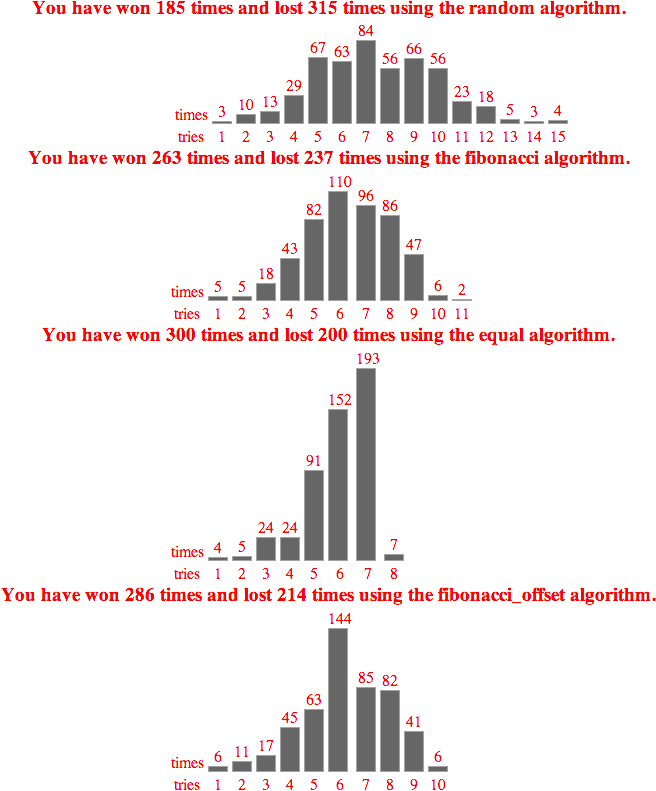# Guess a number between 1 and 100 in 7 or fewer tries

A number is randomly chosen between 1 and 100. The “guesser” is told at each guess whether their guess is correct, too high, or too low.

Four methods tested with the sequence used to successfully guess the number “1.”

**Random** Starts with a random number and draws a random guess from the range implied by the results of each round.
`Guessing: 65, 41, 32, 18, 14, 9, 3, 1`

**Equal Division** Starts with 50 and each time guesses the median value from the range implied by the results of each round.
`Guessing: 50, 25, 13, 7, 4, 2, 1`

**Fibonacci Division** Starts with 50 and each time guesses the golden ratio value from the range implied by the results of each round. In other words, divides by 1.618033989 instead of by 2. So either 32 or 81 will be it’s second round guess.
`Guessing: 50, 32, 21, 14, 10, 7, 5, 4, 3, 2, 1`

**Fibonacci Offset Division** Starts with 50 and each time guesses the golden ratio value based on whether they were too high or too low. So a guess of 50 that was “Too Low” would then choose 81. A guess of 50 that was “Too High” would choose 19.
`Guessing: 50, 19, 7, 3, 1`

I expected that Equal Division would be the winner but wanted to play around with some other options just to see. Here’s the distribution of how many tries it took to guess the correct number over 500 iterations using each of the different methods.

The condition for a win is guessing in fewer tries than the base-2 logarithm of the range (in this case the range is 100 and the base-2 logarithm of 100 is 6).Gregg Hilferding